# Arithmetic progression - problems

1. Loan 5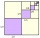Abdul takes a loan of 200000 from Ali and agrees to repay in number of instalment, each instalment begin with the 2nd exceeding the previous one by 1000, if the first instalment is 500, find how many instalment will be necessary to be wipe out the loan? C
2. NumbersDetermine the number of all positive integers less than 4183444 if each is divisible by 29, 7, 17. What is its sum?
3. Ravens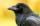The tale of the Seven Ravens were seven brothers, each of whom was born exactly 2.5 years after the previous one. When the eldest of the brothers was 2-times older than the youngest, mother all curse. How old was seven ravens brothers when their mother cur
4. Octahedron - sumOn each wall of a regular octahedron is written one of the numbers 1, 2, 3, 4, 5, 6, 7 and 8, wherein on different sides are different numbers. For each wall John make the sum of the numbers written of three adjacent walls. Thus got eight sums, which also.
5. n-gon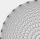Gabo draw n-gon, which angles are consecutive members of an arithmetic sequence. The smallest angle is 70° biggest 170°. How many sides have Gabo's n-gon?
6. SequenceIn the arithmetic sequence is a1=-1, d=4. Which member is equal to the number 203?
7. Mailbox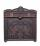Mailbox is opening at regular intervals 3 times a day. The first time is opened at 8:00 and the last at 19:00. Calculate hours when mailbox is opened during day.
8. AVG of INT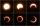What is the average of the integers from 9 throuht 52 inclusive?
9. Cinema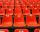Cinema auditorium is built for 3300 people. The first row is planned for 36 seats and each next gradually 4 more. How many rows of seats will have auditorium?
10. LinesIn how many points will intersect 14 different lines, where no two are parallel?
11. Angles in a triangleThe angles of the triangle ABC make an arithmetic sequence with the largest angle γ=83°. What sizes have other angles in a triangle?
12. Series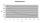Andrew wrote this series of numbers: 1,3,7,15, .... n. Which number in the series does not belong? ?
13. SequenceIn the arithmetic sequence is given: Sn=2304, d=2, an=95 Calculate a1 and n.
14. Gauss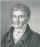Help little C.F. Gauss sum all the integers from 1 to 400.
15. Workman - shift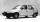The worker produces 300 components per shift. How many components would be produced in 18 shift, if his performance gradually increased every shift by 3 components?
16. SequenceWrite the first 7 members of an arithmetic sequence: a1=-3, d=6.
17. Rope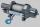How many meters of rope 10 mm thick will fit on the bobbin diameter of 200 mm and length 350 mm (central mandrel have a diameter 50 mm)?
18. Angle in RTDetermine the size of the smallest internal angle of a right triangle whose sides constitutes sizes consecutive members of arithmetic progressions.
19. Sequence 2Write the first 5 members of an arithmetic sequence a11=-14, d=-1
20. Sequence 3Write the first 5 members of an arithmetic sequence: a4=-35, a11=-105.

Do you have an interesting mathematical problem that you can't solve it? Enter it, and we can try to solve it.

To this e-mail address, we will reply solution; solved examples are also published here. Please enter e-mail correctly and check whether you don't have a full mailbox.